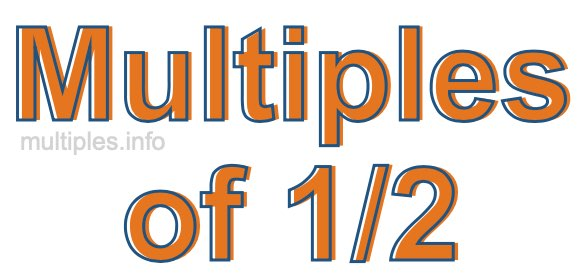Multiples of 1/2We define the multiples of 1/2 as the product of any integer multiplied by 1/2. Therefore, to create a list of multiples of 1/2 we start by multiplying 1/2 by one, then we multiply 1/2 by two, then we multiply 1/2 by three, and so on.

To multiply a fraction by an integer, we leave the denominator alone and multiply the numerator by the integer. It is impossible to give you a list of all multiples of 1/2 because the list goes on forever. However, here is the math to calculate the beginning list of multiples of 1/2.

1/2 × 1 = 1/2

1/2 × 2 = 2/2

1/2 × 4 = 3/2

1/2 × 4 = 4/2

1/2 × 5 = 5/2

1/2 × 6 = 6/2

1/2 × 7 = 7/2

1/2 × 8 = 8/2

1/2 × 9 = 9/2

1/2 × 10 = 10/2

1/2 × 11 = 11/2

1/2 × 12 = 12/2

We have simplified the fractions above for you. Below are the multiples of 1/2 in their lowest possible terms:

1/2 = 1/2

2/2 = 1

3/2 = 1 1/2

4/2 = 2

5/2 = 2 1/2

6/2 = 3

7/2 = 3 1/2

8/2 = 4

9/2 = 4 1/2

10/2 = 5

11/2 = 5 1/2

12/2 = 6

As we stated above, the list of multiples of 1/2 goes on forever because the product of 1/2 multiplied by any whole number (integer) is a multiple of 1/2. You can also create a list of multiples of 1/2 if you keep adding 1/2 like this:

1/2
1/2 + 1/2 = 2/2
1/2 + 1/2 + 1/2 = 3/2
1/2 + 1/2 + 1/2 + 1/2 = 4/2

Again, the list can go on forever, because you can continue adding 1/2 to the list. Therefore, the list of multiples of 1/2 is ∞ (infinite).

Multiples of Fractions
Do you need the multiples of another fraction? Enter a another fraction below to get the multiples of that fraction.

/

Multiples of 1/3
Go here for the next fraction on our list that we have multiples for.